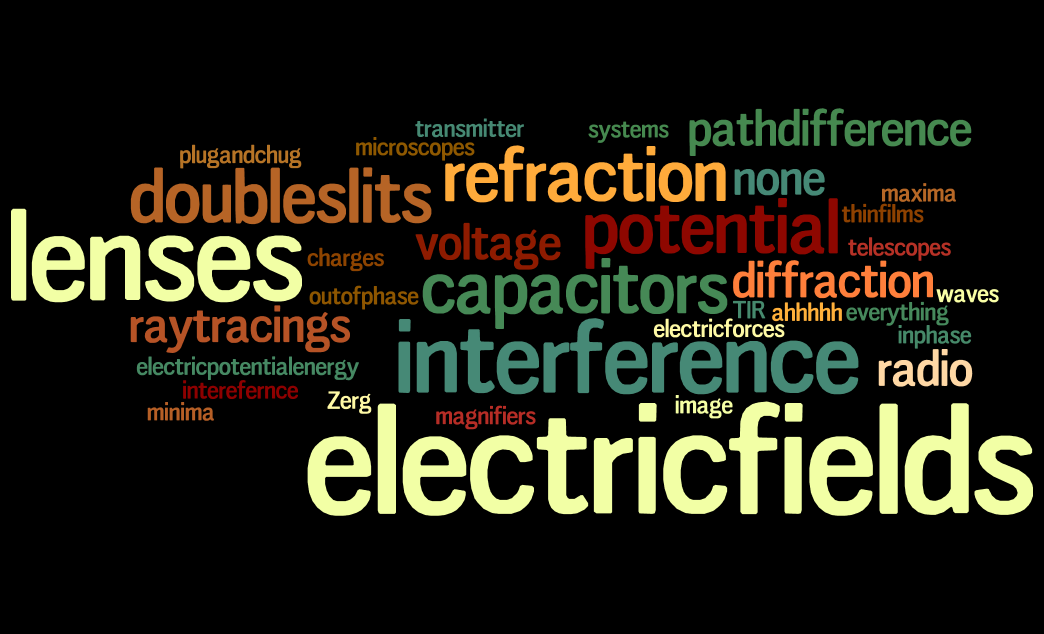# a cool easy problem 4When a voltage of $20 V$ is applied between two ends of a coil, $800 \frac{cal}{s}$ of heat are produced.

What is the resistance of the coil?

• $1 cal = 4.2 J$
×